# Capacitance of Transmission Lines

## Use of Bundled Conductors in Transmission Line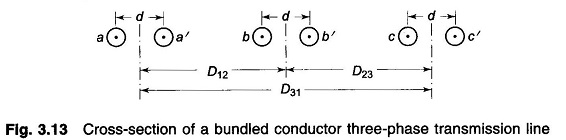Use of Bundled Conductors in Transmission Line: A Bundled Conductors in Transmission Line is shown in Fig. 3.13. The conductors of any one bundle are in parallel, and it is assumed that the charge per bundle divides equally among the conductors of the bundle as D12 ≫ d. Also     D12 – d ≈ …

## Effect of Earth on Capacitance of Transmission LineEffect of Earth on Capacitance of Transmission Line: In calculating the Effect of Earth on Capacitance of Transmission Line, the presence of earth was ignored, so far. The effect of earth on Capacitance of Transmission Line can be conveniently taken into account by the method of images. Method of Images: The electric field of transmission …

## Capacitance of Three Phase Line with Unsymmetrical Spacing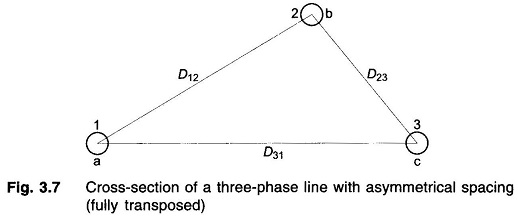Capacitance of Three Phase Line with Unsymmetrical Spacing: Figure 3.7 shows the three identical conductors of radius r of a Capacitance of Three Phase Line with Unsymmetrical Spacing. It is assumed that the line is fully transposed. As the conductors are rotated cyclically in the three sections of the transposition cycle, correspondingly three expressions can …

## Capacitance of Three Phase Transmission Line with Equilateral Spacing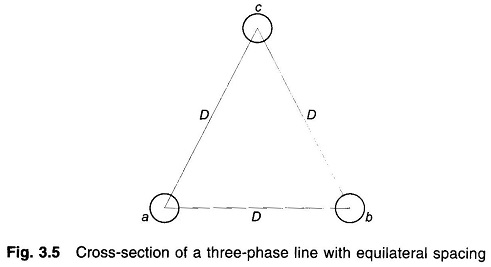Capacitance of Three Phase Transmission Line with Equilateral Spacing: Figure 3.5 shows a Capacitance of Three Phase Transmission Line with Equilateral Spacing composed of three identical conductors of radius r placed in equilateral configuration. Using Eq. (3.2) we can write the expressions for Vab and Vac as Adding Eqs. (3.8) and (3 9), we get …

## Potential Difference between a and b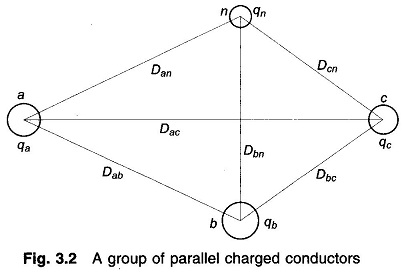Potential Difference between a and b: Figure 3.2 shows a group of parallel charged conductors. It is assumed that the conductors are far removed from the ground and are sufficiently removed from each other, i.e. the conductor radii are much smaller than the distances between them. The spacing commonly used in overhead power transmission lines …

## Electric Field Inside a Conductor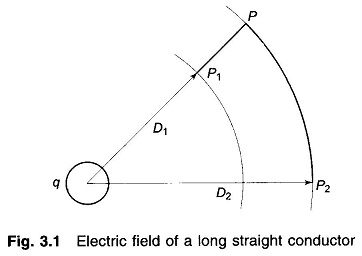Electric Field Inside a Conductor: Imagine an infinitely long straight conductor far removed from other conductors (including earth) carrying a uniform charge of q coulomb/metre length. By symmetry, the equipotential surfaces will be concentric cylinders, while the lines of electrostatic stress will be radial. The Electric Field Inside a Conductor at a distance y from …

Scroll to Top